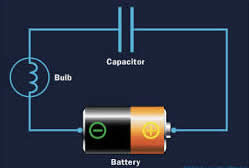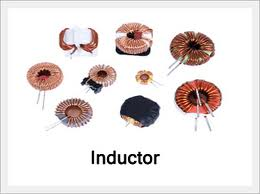# Difference between Capacitor and Inductor

##### Key Difference: Capacitors and inductors are two passive energy storing devices. In capacitors, energy is stored in their electric field. However, in inductors energy is stored in their magnetic field.Capacitor is a device that is used to store an electric charge. It is basically an arrangement of conductors. Due to its characteristics, a capacitor is widely employed in the formation of electronic circuits. A capacitor stores electrical energy directly as an electrostatic field is created between two metal "plates". A capacitor is generally constructed using two metal plates or metal foils separated by an insulator called a dielectric material. Any non-conducting substance can be used as a dielectric material. However, porcelain, mylar, teflon, mica, cellulose are generally preferred. A capacitor is defined by the type of dielectric and electrode material selected. It also defines the application of the capacitor. The dielectric material is the main substance that helps in storing the electrical energy.

The value of capacitance is determined by –

The size of the plates,

The distance between them,

The type of dielectric material used.Inductor is a passive electronic component that can store electrical energy in the form of magnetic energy. It uses a conductor that is wound into a coil. On the flow of electricity into the coil from the left to the right, a magnetic field gets generated in the clockwise direction. Whenever voltage is applied across an inductor, a current starts to flow. The current does not rise instantly. However, it increases gradually over time. The relationship of voltage to current vs. time gives rise to a property known as inductance. The current creates a magnetic field and due to this magnetic field, electric current is stored for a short interval of time. The electric current drops when the magnetic field around the coil collapses.

Comparison between Capacitor and Inductor:

 Capacitor Inductor Definition In capacitors, energy is stored in their electric field. In inductors, energy is stored in their magnetic field. Uses High Voltage Electrolytic used in power supplies. Axial Electrolytic - lower voltage smaller size for general purpose where large capacitance values are needed. High Voltage disk ceramic - small size and capacitance value, excellent tolerance characteristics. Metalised Polypropylene - small size for values up to around 2µF good reliability. Sub−miniature Multi layer ceramic chip (surface mount) capacitor. Relatively high capacitance for size achieved by multiple layers. Effectively several capacitors in parallel. Inductors are widely used in AC application like radio, TV, etc. Chokes – The property of inductor is used in power supply circuits where AC mains supply needs to be converted to a DC supply. Energy store – It is used to create the spark that ignites the petrol in automobile engines. Transformers – Inductors with a sharing magnetic path can be combined to form a transformer. Unit of measurement Capacitance is measured in units called farads (abbreviated F). It is equal to and is equal to a [Ampere-second/Volt].  Since an [Ampere] is a [Coulomb/second], we can also say that a [F]=[C/V]. The value of an Inductor is called Inductance and is measured in Henries. It is actually the SI unit of Inductance. It is equal to a [Volt-second/Ampere]. Types Three major types of capacitors are ceramic, electrolytic, and tantalum: Ceramic capacitors - They are quite smaller in size and value, ranging from a few Pico Farads to 1 µF. Electrolytic capacitors - They resemble small cylinders and range in value from 1 µF to several Farads. Tantalum capacitors – They are quite similar in size to ceramic. However, they can hold more charge, up to several hundred µF. They tend to be accurate and stable. Three major types of inductors are Coupled inductors, Multi-Layer Inductors, Ceramic Core Inductor and Molded Inductors: Coupled inductors- They show magnetic flux that is dependent on other conductors to which they are linked. Multi-Layer Inductors - This particular type of inductor consists of a layered coil, wound multiple times around the core. Due to these multiple layers and the insulation between them, multi-layer inductors have a comparatively high inductance level. Ceramic Core Inductors - a ceramic core inductor possess a dielectric ceramic core. It means that it is not capable of storing a lot a lot of energy but has very low distortion and hysteresis. Molded Inductors - These types of inductors are molded using a plastic or ceramic insulation. Relationship between voltage and current in linear circuit Voltage lags behind Current by  π/2 Current lags behind Voltage  by π/2 Short circuit A capacitor acts as a short circuit for Alternating Current. An Inductor is equivalent to a short circuit to Direct Current. Characteristics Capacitors connected in parallel combine like resistors in series Capacitors in series combine like resistors in parallel Inductors in parallel combine like resistors in parallel Inductor in series combine like resistor in series